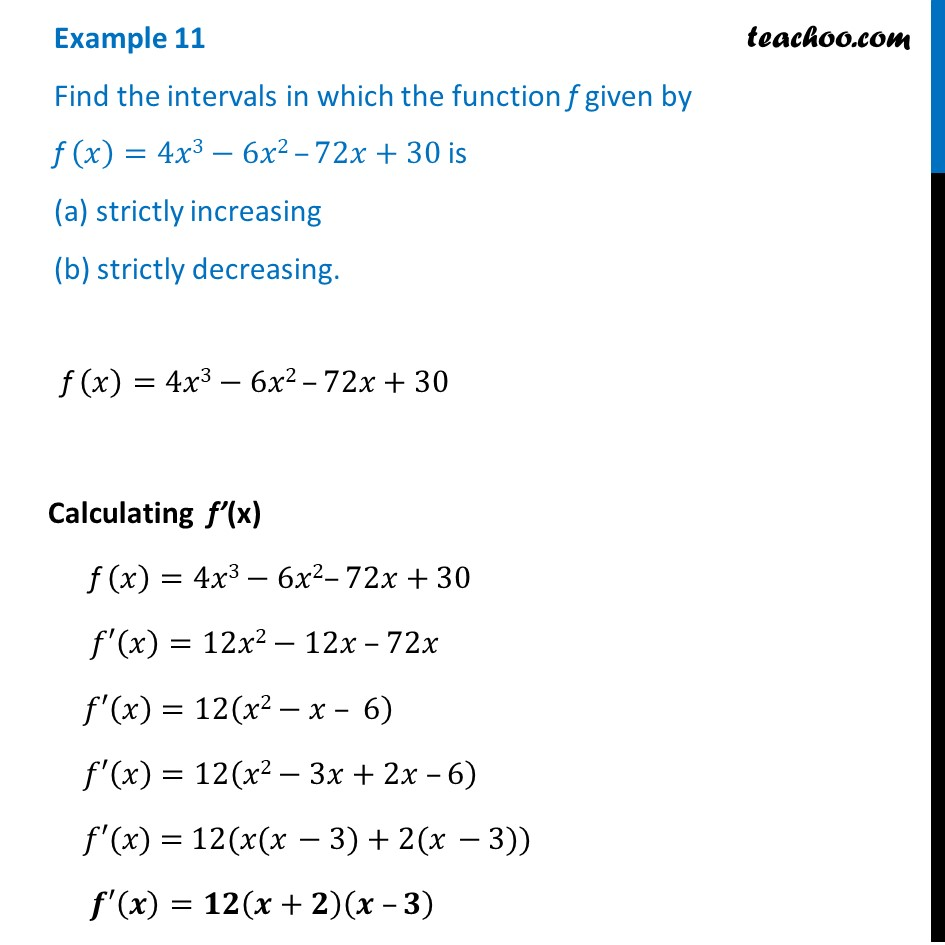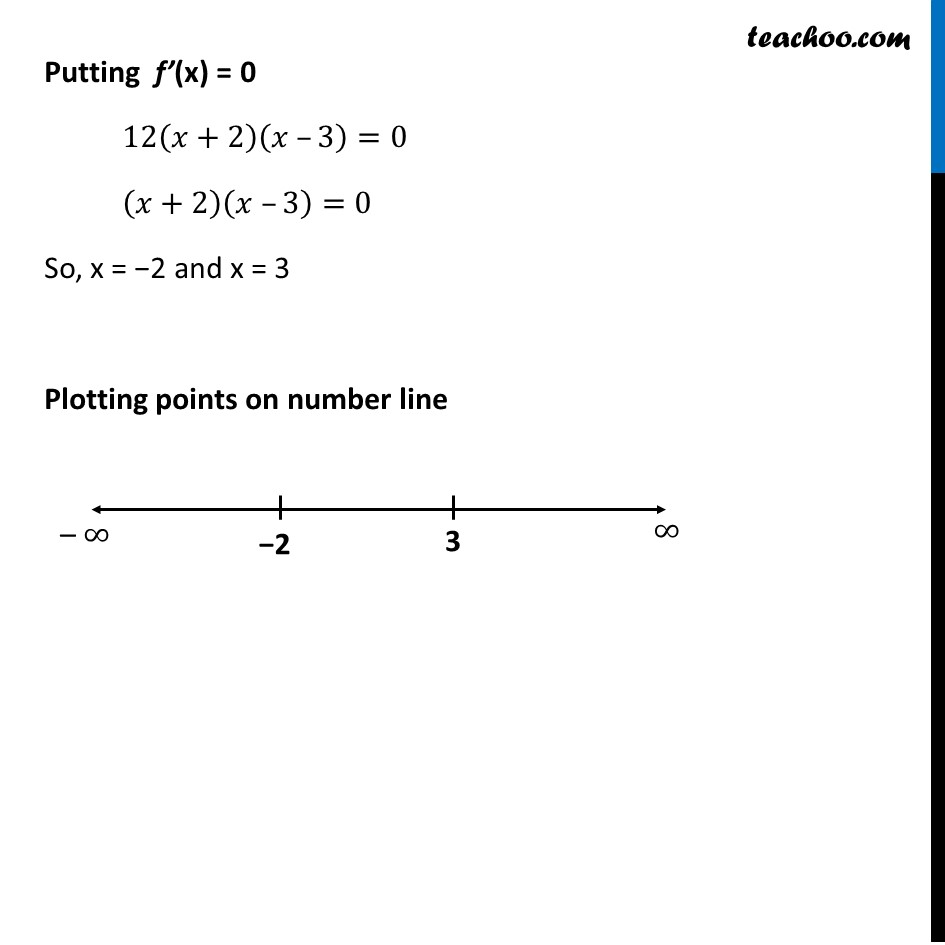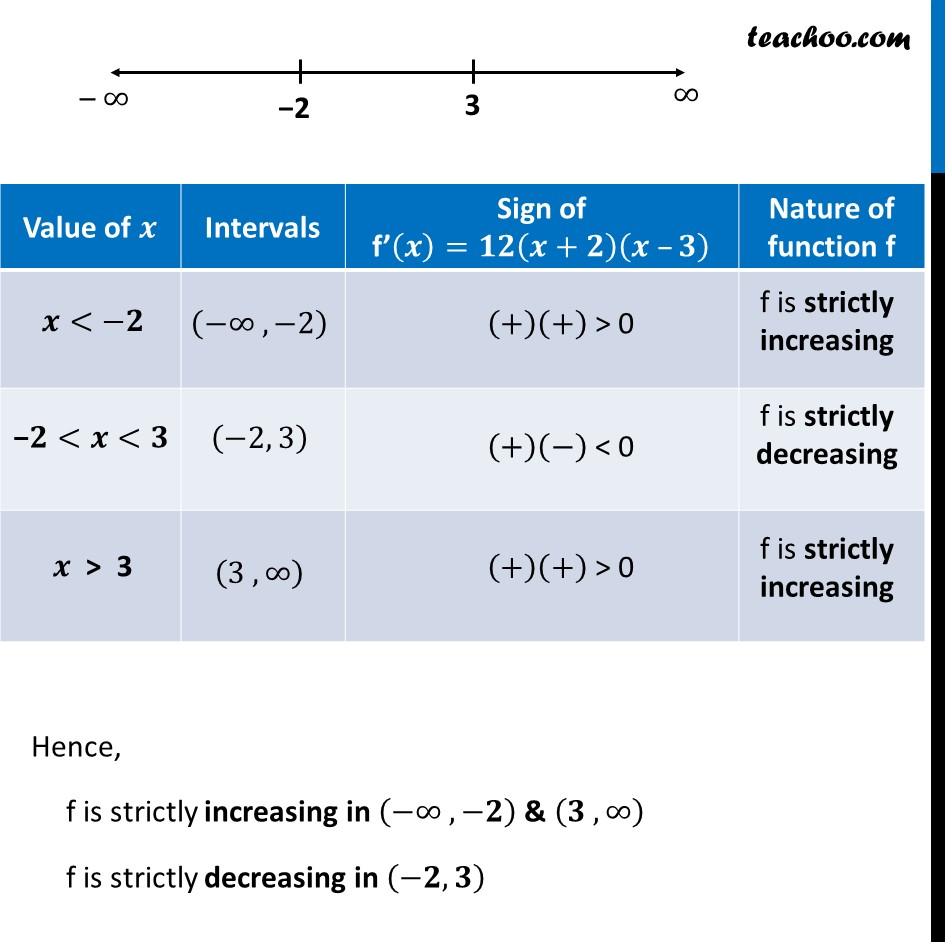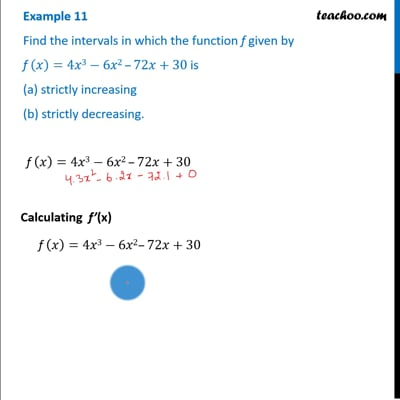Examples

Chapter 6 Class 12 Application of Derivatives
Serial order wiseThis video is only available for Teachoo black users

Learn in your speed, with individual attention - Teachoo Maths 1-on-1 Class

### Transcript

Example 11 Find the intervals in which the function f given by f (𝑥)=4𝑥3−6𝑥2 –72𝑥+30 is (a) strictly increasing (b) strictly decreasing. f (𝑥)=4𝑥3−6𝑥2 –72𝑥+30 Calculating f’(x) f (𝑥)=4𝑥3−6𝑥2–72𝑥+30 𝑓′(𝑥)=12𝑥2−12𝑥 –72𝑥 𝑓′(𝑥) =12(𝑥2−𝑥 – 6) 𝑓′(𝑥) =12(𝑥2−3𝑥+2𝑥 –6) 𝑓′(𝑥) = 12(𝑥(𝑥 − 3) + 2(𝑥 − 3)) 𝒇′(𝒙)=𝟏𝟐(𝒙+𝟐)(𝒙 –𝟑) Putting f’(x) = 0 12(𝑥+2)(𝑥 –3)=0 (𝑥+2)(𝑥 –3)=0 So, x = −2 and x = 3 Plotting points on number line Hence, f is strictly increasing in (−∞ ,−𝟐) & (𝟑 ,∞) f is strictly decreasing in (−𝟐, 𝟑)